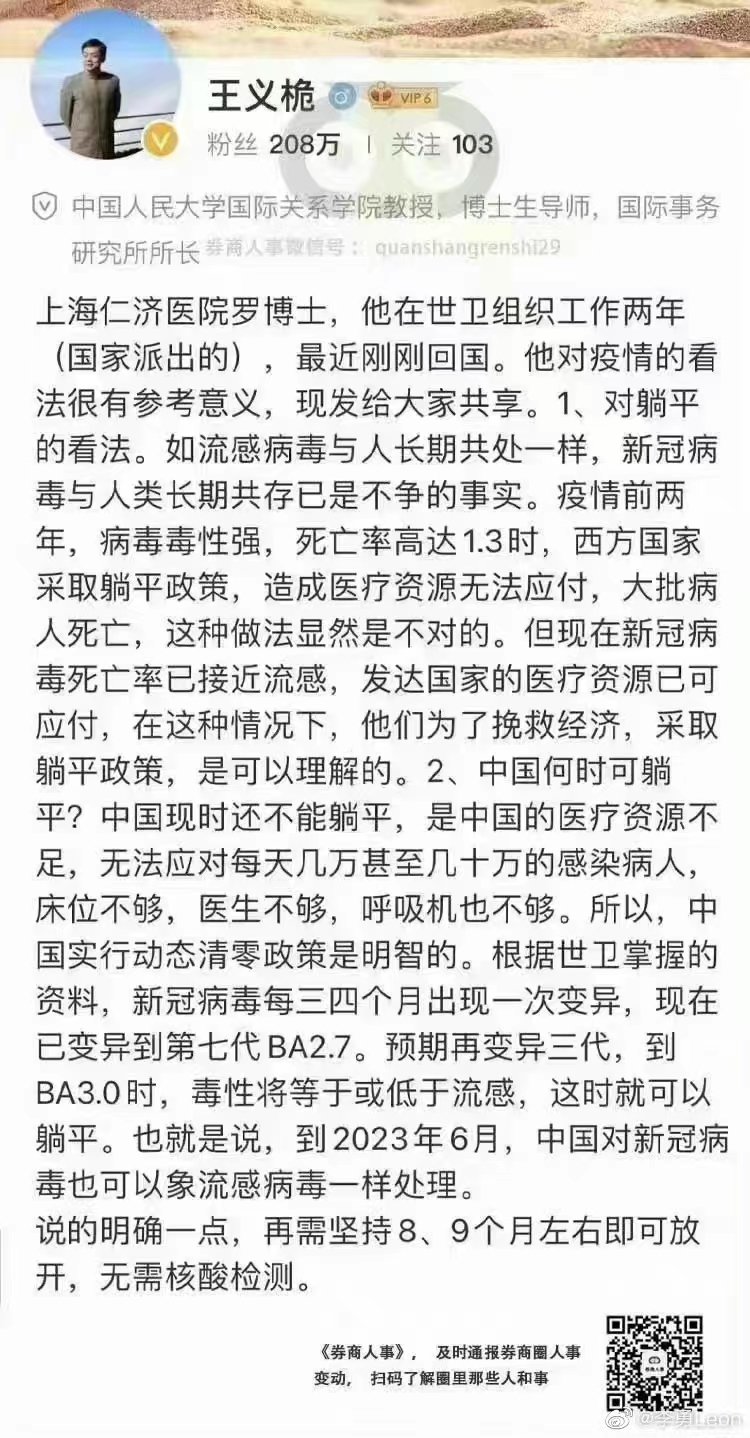# ch3co3h

## 复数概念的另一种引入方式



$$+c <x2, y2> = <x1+x2, y1+y_2>$$

$$a+b\text{i}+_cc+d\text{i}=(a+c)+(b+d)\text{i}$$

$$\timesc<x2,y2>=<x1x2,y1y_2>$$

$$\begin{bmatrix}x2\y2\end{bmatrix}= \begin{bmatrix}r\cos(\theta+\phi)\r\sin(\theta+\phi)\end{bmatrix}= \begin{bmatrix}\cos\phi & -\sin\phi\\sin\phi&\cos\phi\end{bmatrix} \begin{bmatrix}x1\y1\end{bmatrix}$$

$$\timesc<x2,\space y2>=<x1x2-y1y2,\space x1y2+x2y_1>$$

$$(a+b\text{i})\times(c+d\text{i})=ac-bd+(ad+bc)\text{i}$$

 元组是计算机科学中的术语，指同类型元素的有序排列。元组与集合的差异是：元组中元素可以重复，集合不可以；元组中元素有顺序，集合没有。

## 写数学公式的麻烦

$$\begin{eqnarray} \nabla \times \mathbf H =\mathbf J+\frac{\partial \mathbf D}{\partial t}\newline \nabla \times \mathbf D=-\frac{\partial \mathbf B}{\partial t}\newline \nabla \cdot \mathbf B=0\newline \nabla \cdot \mathbf D=\rho \end{eqnarray}$$

i=√-1

$$\text i=\sqrt {-1}$$

\nabla \times \mathbf H =\mathbf J+\frac{\partial \mathbf D}{\partial t}\\
\nabla \times \mathbf D=-\frac{\partial \mathbf B}{\partial t}\\
\nabla \cdot \mathbf B=0\\
\nabla \cdot \mathbf D=\rho


nabla times bold H  = bold J + frac { partial bold D } { partial t } newline
nabla times bold E = - frac { partial bold B } { partial t } newline
nabla cdot bold B=0 newline
nabla cdot bold D= %rho


Imaginary number unit: \$$\text {i} = \sqrt {-1} \$$


Imaginary number unit: $\text {i} = \sqrt {-1}$


Imaginary number unit: $\text {i} = \sqrt {-1}$

MathJax = {
tex: {
inlineMath: [['$', '$'], ['\$$', '\$$']]
}
};


n!=n*(n-1)*...*1; 另外 0!=1。

frac (n)
if n=0 return 1;
return n*frac(n-1);


$$v=v(t)$$

real v (real t);
int upper (real x);


## 【百篇原创】承受生命中的大起大落

2016 年 03 月 28 日，是我发布第一篇文章《传说中的H5到底是什么》的日子。随后经过约两年，我的号拿到原创标签权限，于 2018 年 01 月 10 日发布了第一篇带原创标签文章《双注输入法的诞生》。5 年之后，算上此文，我的原创内容数量终于来到 100。这是个值得纪念的时间点，但看到标题的您或许早已猜到，我目前实在是没有办法对这个事情有任何兴奋。

γνῶθι σεαυτόν

## 驯化

《名著看不进克怎么办》是和菜头 10 月 10 日发布的一篇文章（标题没有错别字，「克」是「去」的方言替代）。当时我在看文章评论时发现一条特别有意思的，一个激动就截了图。